# 使用全卷积神经网络FCN，进行图像语义分割详解(附带Tensorflow详解代码实现)

2020/07/25 14:09

## 一.导论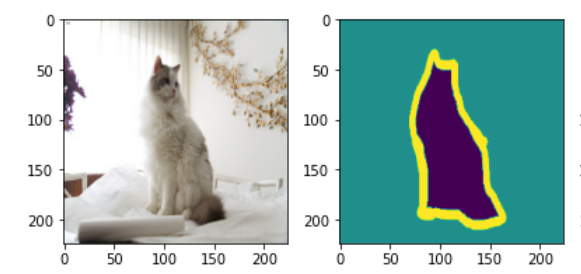而在2015年出来的FCN，全卷积神经网络完美地解决了这个问题，将曾经mean IU(识别平均准确度)只有百分之40的成绩提升到了百分之62.2（在Pascal VOC数据集上跑的结果，FCN论文上写的），像素级别识别精确度则是90.2%。这已经是一个相当完美的结果了，几乎超越了人类对图像进行区分，分割的能力。如上图所示，小猫被分割为了背景，小猫，边缘这三个部分，因此图像当中的每一个像素最后只有三个预测值，是否为小猫，背景，或者边缘。全卷积网络要做的就是这种进行像素级别的分类任务。那么这个网络是如何设计和实现的呢？

## 二.网络的实现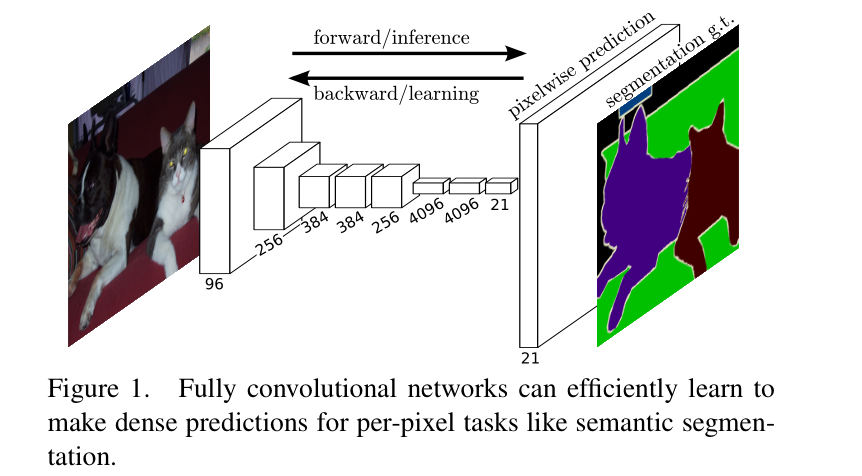## 三.图像的上采样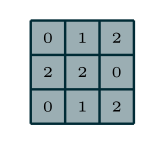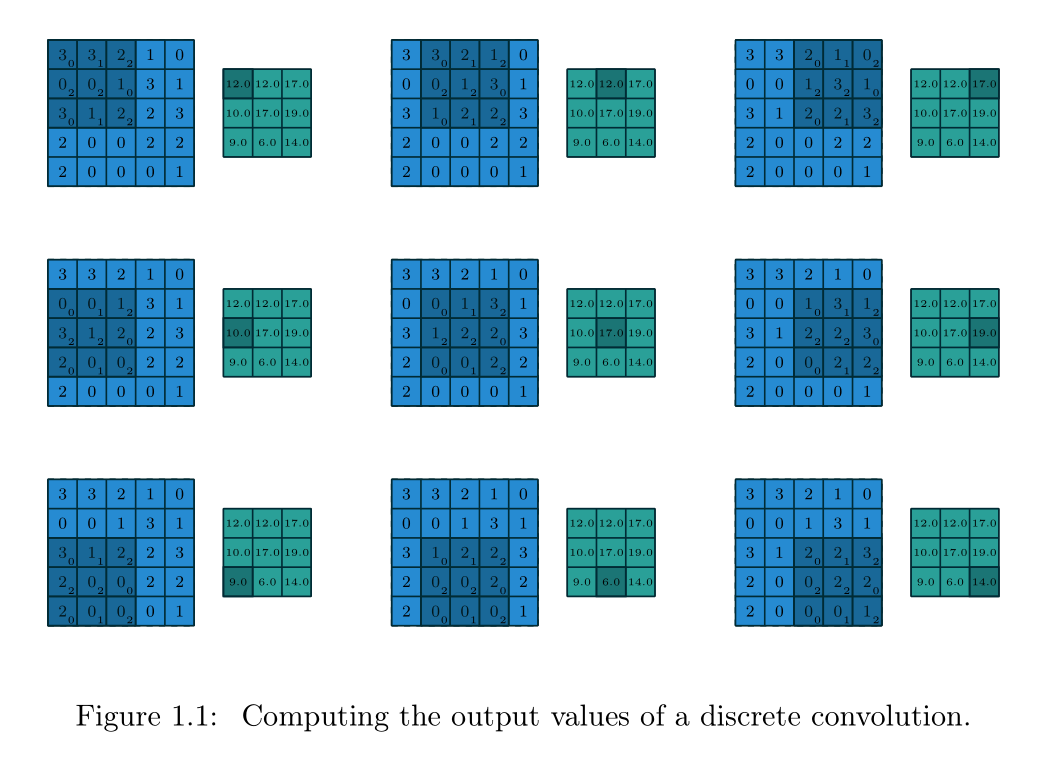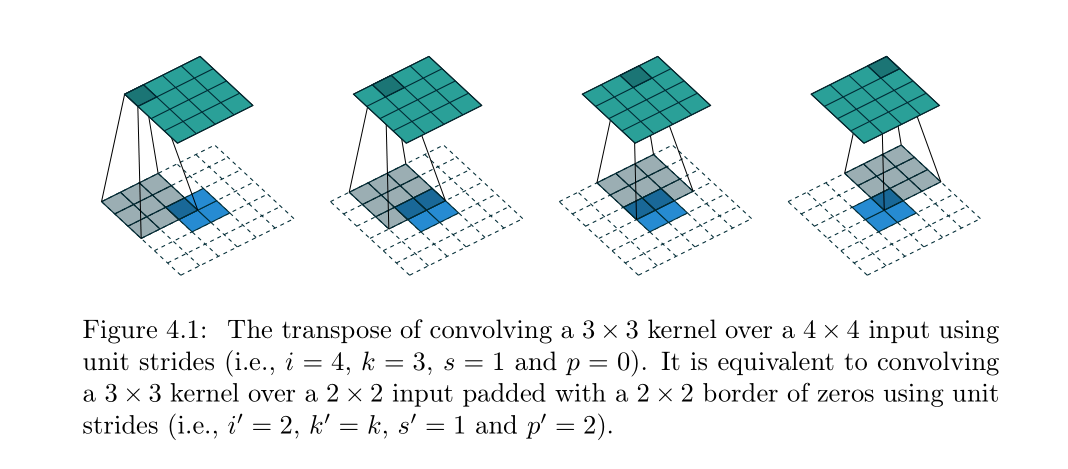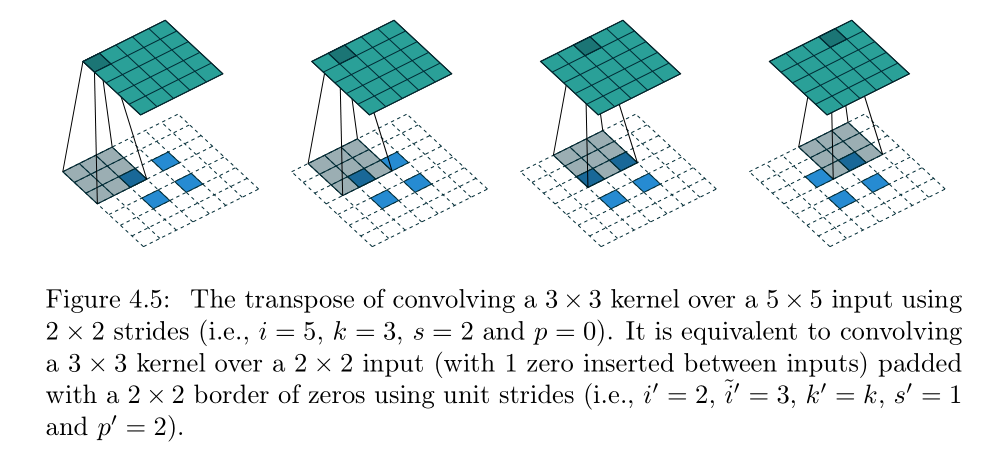这样咱们的反卷积就完成了。那么什么是1*1卷积呢？

## 四.1*1卷积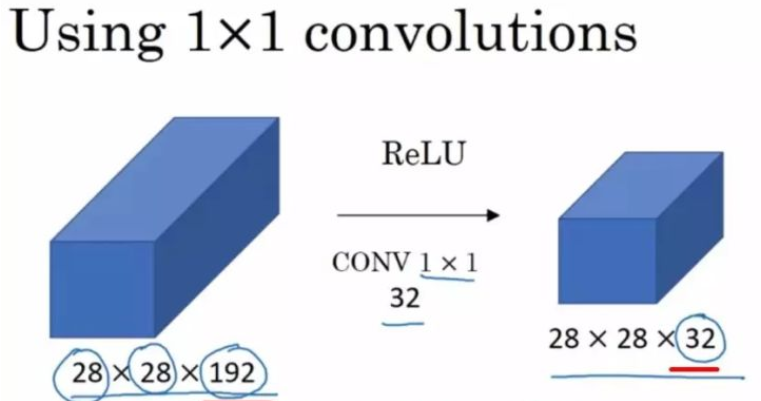原本的特征图长宽为28，channel为192，我们可以通过这种卷积，使用32个卷积核将28*28*192变成一个28*28*32的特征图。在使用1*1卷积时，得到的输出长款保持不变，channel数量和卷积核的数量相同。可以用抽象的3d立体图来表示这个过程：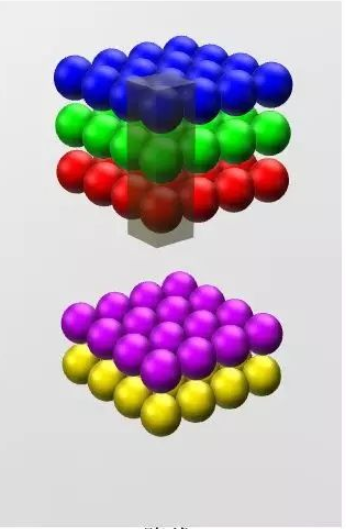因此我们可以通过控制卷积核的数量，将数据进行降维或者升维。增加或者减少channel，但是feature map的长和宽是不会改变的。我们在全卷积神经网络（FCN）正向传播，下采样的最后一步（可以查看本博客的第一张图片）就是将一个N*N*4096的特征图变成了一个N*N*21的特征图。

## 五.全卷积神经网络的跳级实现（skip）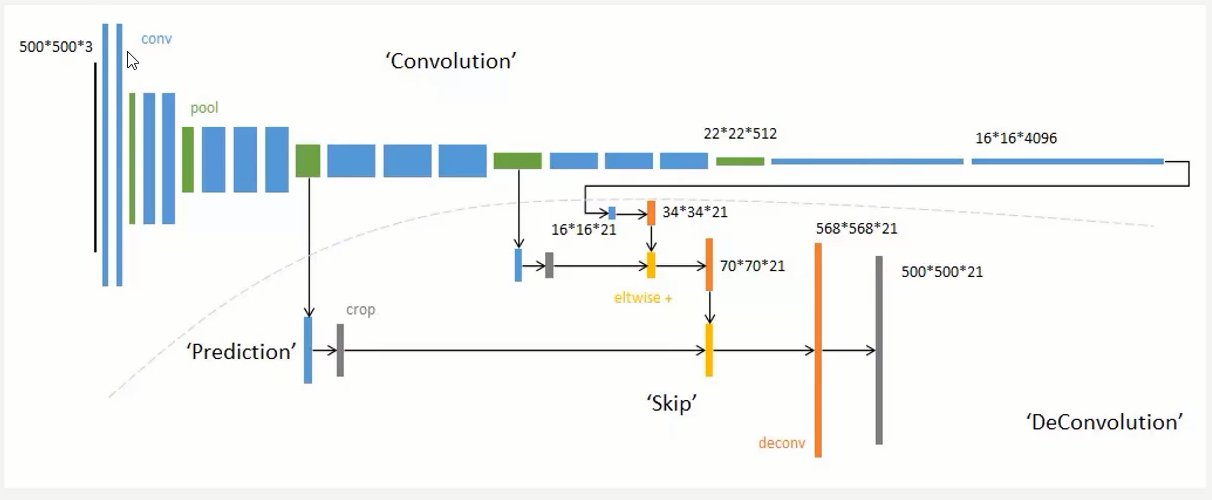作者最先提出的跳级连接是把第五层的输出进行上采样，然后和池化层4的预测相结合起来，最后得到原图的策略，这个策略叫做FCN-16S,之后又尝试了和所有池化层结合起来预测的方法叫做FCN-8S,发现这个方法准确率是最高的。如下图所示：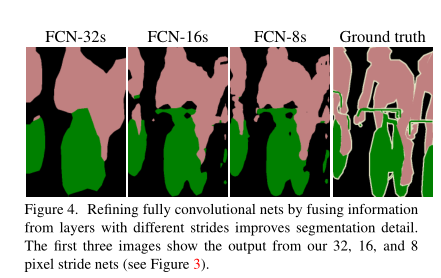Groud Truth表示原始图像的人为标注，前面的都是神经网络做出的预测。跳级连接，我们这类给出的原图的大小是500*500*3,这个尺寸无所谓，因为全卷积神经网络可接受任意尺寸大小的图片。我们首先从前面绿色刚刚从池化层做完maxpool的特征图上做一次卷积然后，然后再把下一个绿色的特征图做卷积，最后把16*16*21，已经做完1*1卷积的输出，把这个三个输出相加在一起，这样就实现了跳级（skip）输入的实现，再把这几个输入融合之后的结果进行上采样，得到一个568*568*21的图，将这个图通过一个softmax层变成500*500*21的特征图，因此图像的长宽和原图一模一样了，每一个像素点都有21个概率值，表示这个像素点属于某个类别的概率，除了和原图的channel不同之外没啥不同的。

## 六.Tensorflow代码实现全卷积神经网络

import tensorflow as tf
import matplotlib.pyplot as plt
import numpy as np
import os

import glob
images=glob.glob(r"F:\UNIVERSITY STUDY\AI\dataset\FCN\images\*.jpg")
#然后读取目标图像
anno=glob.glob(r"F:\UNIVERSITY STUDY\AI\dataset\FCN\annotations\trimaps\*.png")

glob库可以用于读取本地的图片并用来制作每一个batch的数据，我把数据集放在了F:\UNIVERSITY STUDY\AI\dataset\FCN\，这个文件夹下。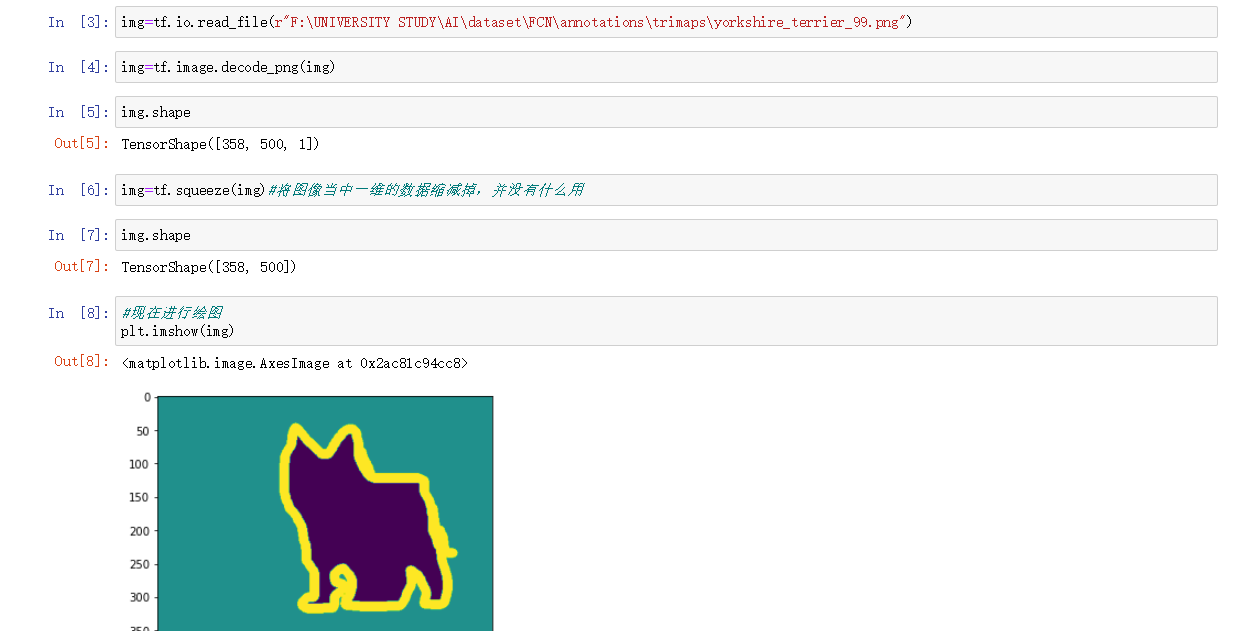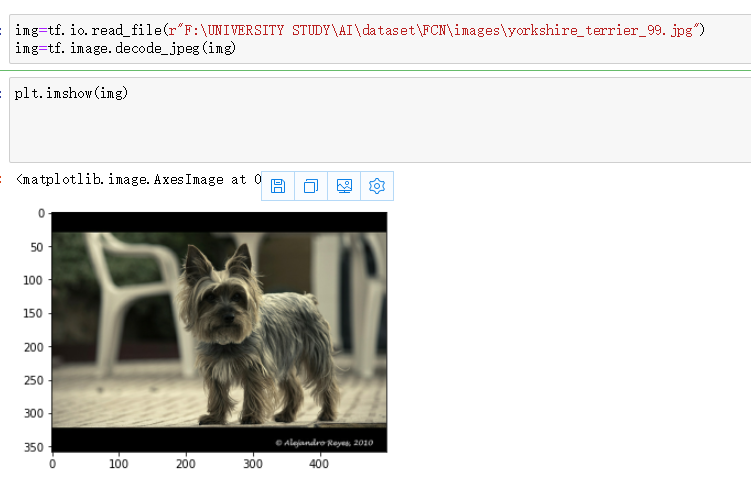然后制作dataset，batch数据，以及读取图片文件的函数，包括png和jpg分别进行解析为三维矩阵：

#现在对读取进来的数据进行制作batch
np.random.seed(2019)
index=np.random.permutation(len(images))

images=np.array(images)[index]
anno=np.array(anno)[index]
#创建dataset
dataset=tf.data.Dataset.from_tensor_slices((images,anno))
test_count=int(len(images)*0.2)
train_count=len(images)-test_count
data_train=dataset.skip(test_count)
data_test=dataset.take(test_count)

img=tf.image.decode_jpeg(img,channels=3)
return img

img=tf.image.decode_png(img,channels=1)
return img

#现在编写归一化的函数
def normal_img(input_images,input_anno):
input_images=tf.cast(input_images,tf.float32)
input_images=input_images/127.5-1
input_anno-=1
return input_images,input_ann

#加载函数
input_image=tf.image.resize(input_image,(224,224))
input_anno=tf.image.resize(input_anno,(224,224))
return normal_img(input_image,input_anno)

#现在开始batch的制作
BATCH_SIZE=3#根据显存进行调整
data_train=data_train.repeat().shuffle(100).batch(BATCH_SIZE)

data_test=data_test.batch(BATCH_SIZE)

conv_base=tf.keras.applications.VGG16(weights='imagenet',
input_shape=(224,224,3),
include_top=False)
#现在创建子model用于继承conv_base的权重，用于获取模型的中间输出
#使用这个方法居然能够继承，而没有显式的指定到底继承哪一个模型，确实神奇
#确实是可以使用这个的，这个方法就是在模型建立完之后再进行的调用
#这样就会继续自动继承之前的网络结构
#而如果定义
sub_model=tf.keras.models.Model(inputs=conv_base.input,
outputs=conv_base.get_layer('block5_conv3').output)

#现在创建多输出模型,三个output
layer_names=[
'block5_conv3',
'block4_conv3',
'block3_conv3',
'block5_pool'
]

layers_output=[conv_base.get_layer(layer_name).output for layer_name in layer_names]

#创建一个多输出模型，这样一张图片经过这个网络之后，就会有多个输出值了
#不过输出值虽然有了，怎么能够进行跳级连接呢？
multiout_model=tf.keras.models.Model(inputs=conv_base.input,
outputs=layers_output)

multiout_model.trainable=False

inputs=tf.keras.layers.Input(shape=(224,224,3))
#这个多输出模型会输出多个值，因此前面用多个参数来接受即可。
out_block5_conv3,out_block4_conv3,out_block3_conv3,out=multiout_model(inputs)
#现在将最后一层输出的结果进行上采样,然后分别和中间层多输出的结果进行相加，实现跳级连接
#这里表示有512个卷积核，filter的大小是3*3
x1=tf.keras.layers.Conv2DTranspose(512,3,
strides=2,
activation='relu')(out)
#上采样之后再加上一层卷积来提取特征
activation='relu')(x1)
#与多输出结果的倒数第二层进行相加，shape不变
#x2进行上采样
x2=tf.keras.layers.Conv2DTranspose(512,3,
strides=2,
activation='relu')(x2)
#直接拿到x3，不使用
#x3进行上采样
x3=tf.keras.layers.Conv2DTranspose(256,3,
strides=2,
activation='relu')(x3)
#增加卷积提取特征
#x4还需要再次进行上采样，得到和原图一样大小的图片，再进行分类
x5=tf.keras.layers.Conv2DTranspose(128,3,
strides=2,
activation='relu')(x4)
#继续进行卷积提取特征
#最后一步，图像还原
preditcion=tf.keras.layers.Conv2DTranspose(3,3,
strides=2,
activation='softmax')(x5)

model=tf.keras.models.Model(
inputs=inputs,
outputs=preditcion
)

model.compile(
loss='sparse_categorical_crossentropy',
metrics=['acc']#这个参数应该是用来打印正确率用的，现在终于理解啦啊
)

model.fit(data_train,
epochs=1,
steps_per_epoch=train_count//BATCH_SIZE,
validation_data=data_test,
validation_steps=train_count//BATCH_SIZE)

Train for 1970 steps, validate for 1970 steps
1969/1970 [============================>.] - ETA: 1s - loss: 0.3272 - acc: 0.8699WARNING:tensorflow:Your input ran out of data; interrupting training. Make sure that your dataset or generator can generate at least steps_per_epoch * epochs batches (in this case, 1970 batches). You may need to use the repeat() function when building your dataset.
1970/1970 [==============================] - 3233s 2s/step - loss: 0.3271 - acc: 0.8699 - val_loss: 0.0661 - val_acc: 0.8905### 作者的其它热门文章

0
0 收藏

0 评论
0 收藏
0